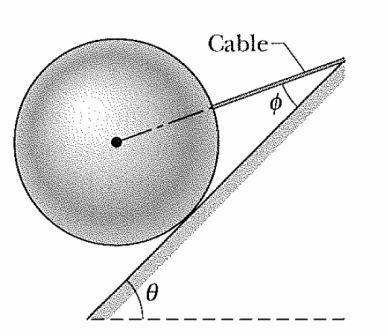# Inclined string on an incline planeIn the figure above, a 10 kg sphere is supported on a frictionless plane inclined at angle $\theta$= 45° from the horizontal. The angle $\phi$ is 25°. Calculate the tension in the cable (in N).

Liked it? try some more

×